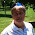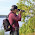## Tuesday, November 18, 2014

### NFL Picks Contest- Week 11 Results and Standings

Here are the Week 11 results and standings in the NFL Picks Contest. Cokeboy sticks with the Packers again this week and rockets to the top!

Here are the current standings;

cokeboy99- 27-(8)+21+34+16+13+20+38+16+51+43 = 271 pts.
OneAndDone- 27+17+17+34+41+5+31+38+16+24+17 = 267 pts.
MOJO-  27+17+17+29+16 +24+32+37+16+34+17 = 266 pts.
lightning36- 27+17+17+29+16+24+31+24+20+34-(15) = 224 pts.
Captain Crunch- 27+17+17+29+16+5+35+38+24+24-(17) = 215 pts.
Vegas Vic- 27+17+21+29+16+5+32-(3)+20+31+17 = 212 pts.

mrben09- 27+17+17+29+16+24+31+37-3+24-(15) = 204 pts.
Music City Momma- 13+17+21+29+16-(7)+32+38-(3)+31+17 = 204 pts.
ggrouchie- 27+17+17+34+41+13+20-(3)+24+24-(15) = 199 pts.
Grange95- (3)+17+21+29+16+24+11+20+16+0+43 = 194 pts.
TwoMinuteWarning- (3)+17+37+29+16-(7)+35+37+20+24-(17) = 188 pts.

Neophyte- 27+17-(19)+0+41+24+21+0+24+24+17 = 176 pts.
M Prosk- (3)+ 0+ 0+34+41+13+11+20+20+24+0 = 160 pts.
Coach- 27+17+21+29+20+24+20-(3)+16-(7)-(20) = 144 pts.
TeamMiRketti- 27+17+17-(3)+16+24+0-(19)+20+34+0 = 133 pts.

Week 11 scores

angerisagift- Carolina (L 17-19) = (2) pts.
MOJO- San Diego (W 13-6) = 17 pts.
Vegas Vic- San Diego (W 13-6) = 17 pts.
Captain Crunch- New Orleans (L 10-27) = (17) pts.
OneAndDone- San Diego (W 13-6) = 17 pts.
mrben09- Denver (L 7-22) = (15) pts.

ggrouchie- Denver (L 7-22) = (15) pts.
Coach- Washington (L 7-27) = (20) pts.
TeamMiRketti-  0 pts.
lightning36- Denver (L 7-22) = (15) pts.
Neophyte- San Diego (W 13-6) = 17 pts.

Music City Momma- San Diego (W 13-6) = 17 pts.
TwoMinuteWarning- New Orleans (L 10-27) = (17) pts.
Grange95- Green Bay (W 53-20) = 43 pts.
M Prosk- 0 pts.
cokeboy99- Green Bay (W 53-20) = 43 pts.

Thanks for playing.

Week 12 picks are due by Saturday at 6pm (CDT). Only Sunday and Monday games are eligible.

Good luck!

1.Dang Broncos ...

2.Green Bay is turning into what Denver was last year. What a machine.

3.Damn, who's the smart guy at the top this week? :-)

1.That would be the "Rocketman."

WTG!

4.Thanks sir! I'm aiming for some Dallas Cowboys gear!! lol